# PROOF: Reproductive Property of the Gamma Distribution / Probability Theory

In this page, we show that the sum of two gamma-distributed random variables, Y:=X1+X2, also follows the gamma distribution: i.e. the gamma distribution has the reproductive property.

## Reproductive Property of the Gamma Distribution

If the two random variables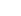and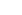follow the gamma distributions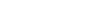and:

(1)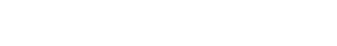then the sum of the two random variables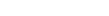follows the gamma distribution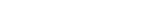:

(2)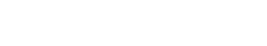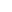### Preparation for the Proof

The following equation holds:

(3)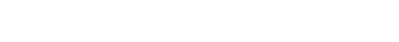If we transform the variable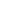in the left side of the above equation (3) into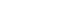, then we obtain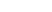,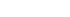, and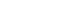, thus

(4)The integration on the last part of the above equation (4) is the beta function, which is written as

(5)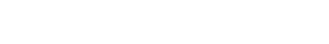using the gamma functions.
Therefore we obtain

(6)### Proof

Generally the PDF of the sum of two random variables,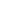, is derived from the convolution of the PDFs,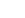and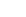:

(7)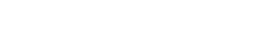If we substitute the PDFs of the gamma distributions (1) into the above convolution (7), we obtain

(8)The integration on the last part of the above equation (8) equals to the equation (3) which is shown in the Preparation, thus we obtain the following equation:

(9)[Q.E.D.]

#### Remark 1

The gamma distribution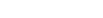with the integer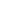and the parameter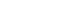implies the Erlang distribution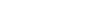. The proof in this page also holds in the Erlang distribution. [Reproductive Property of the Erlang Distribution (in JPN) ]